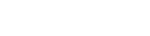# Numbers

Numbers are an important type of data in any computer program. GDScript supports integers and floating point values. And both types may be positive or negative.

We might assign numbers to variables. Then the value may be passed around and changed. If it’s important that the value should not be changed then it should be assigned to a constant.

``````# Integers
var a = 1
var b = -23
var c = 0
var d = 0xA1Fe316 # hexadecimal

# Floats
var x = 1.0
var y = -43.01
var z = 1.3e6

# Constant

``````

Pre-defined constants include: PI, TAU, INF (infinity)

For an exercise, write code to print numbers to the Output window of the Editor.

Ideas to play around with:

• What happens if you try to change the value of a constant?
• Try entering a floating point value with an exponent like 3e6 (3 million)
• What happens if you enter crazy large numbers?
• Does it matter what case you use for hex digits?
• Use the built-in constants to see their output values

Here is a code template for this to get started:

``````extends Node2D

const SPEED = 75
var number = SPEED

# Called when the node enters the scene tree for the first time.
# Print the value to the Output window of the editor
print(number)
number = 86
print(number)
``````

## Enums

Enums are useful for defining several related constants where you don’t care about the value that is automatically assigned by the engine e.g. `enum {COLD, WARM, HOT}`

## A bit more about numbers stored on a computer

Computer chips store data as sequences of 1’s and 0’s in binary. Each value is a bit. Integers are stored as 64 bits. This limits the range of integer values.

If you keep adding 1 to an integer it will reach the maximum positive value (63 1’s), and then, adding another 1 will flip the sign bit (the 64th bit), as all the preceding 1’s carry the new 1 over (in binary arithmetic), giving the maximum negative value. Then we start counting back towards zero and up to the maximum positive value again.

So the number wraps around. Do you think that this is better than having a show-stopping (crash) overflow error?

## Number Wrapping

In a game, it can be useful to have numbers wrap around. For example, a position coordinate along a repeating terrain. Maybe the terrain x-coordinate is mapped as an integer, and the terrain height is drawn up and down as you move along it as a function of x?

But you may want your warrior to traverse the terrain for as long as the game lasts. So, if x is an integer that wraps around, you don’t need to worry about an error occurring when the maximum value of x is reached during the final stages of an e-sports tournament.

## Integers

Integers are ideal for counters, index values, and ID numbers where whole numbers are wanted. Even the most popular multiplayer game is unlikely to run out of unique ID numbers for players using integers. Yeah, but watch out for the bots!

## Floating Point Numbers

Floating point numbers are used a lot for anything else such as amounts, angles, lengths etc. They are not so good as integers for exact comparisons such as `A equals zero` since there are precision errors to consider. For example: 0.0001 looks like a close enough approximation to zero, but it is actually greater than zero in code.

## Conclusion

When mixing numbers in calculations and assignments, casting takes place when the type of number is changed. This can lead to unexpected results unless we take precautions. Look forward to exploring this interesting topic in a future tutorial.

The next topic is: Variables